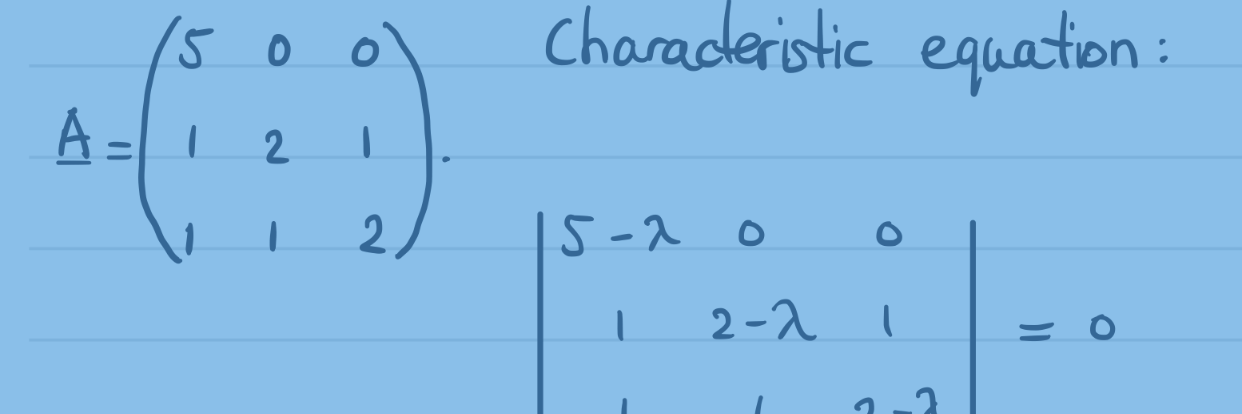# Spaces and dimensions

What do mathematicians and physicists mean with phrases like higher and/or extra dimensions? Let’s travel through a Universe of spaces and dimensions! Continue reading Spaces and dimensions# Complex numbers: an introduction

We will leave the domain of real numbers behind us and start exploring the plane of complex numbers. An introduction to a realm beyond imagination. Continue reading Complex numbers: an introduction# Lab centrifuges and prime numbers

Lab centrifuges are crucial in e.g. coronavirus research. It’s vital the test tubes are balanced. There is an easy method to know if that’s possible. Continue reading Lab centrifuges and prime numbers# The Collatz Conjecture

The Collatz Conjecture is probably one of the easiest to understand problems which hasn’t yet been answered in the history of mathematics. Continue reading The Collatz Conjecture# Proof that the square root of 2 is irrational

We will provide the proof that the square root of 2 is irrational through a proof of contradiction. We will show no valid ratio of integers exists. Continue reading Proof that the square root of 2 is irrational# Finding the normal force in planar non-uniform circular motion using polar coordinates

We find an expression for the normal force on a mass which is in planar non-uniform circular motion using polar coordinates. Continue reading Finding the normal force in planar non-uniform circular motion using polar coordinates# Deriving the volume of the inside of a sphere using spherical coordinates

Using a volume integral and spherical coordinates, we derive the formula of the volume of the inside of a sphere, the volume of a ball. Continue reading Deriving the volume of the inside of a sphere using spherical coordinates# Happy birthday mister Einstein, happy Pi Day to you!

Pi Day is the day on which we commemorate Albert Einstein’s birthday. Also, people celebrate the existence of pi. Here are some cool ways to calculate pi. Continue reading Happy birthday mister Einstein, happy Pi Day to you!# Mirror, mirror, what’s up with the mirror writing?

Ever wondered why sentences, words, and letters always exclusively seem to have their left and right reversed in the mirror, while they are almost never projected upside down? Probably, because mirrors do something else than you would expect. For starters, mirrors don’t reverse left and right. Continue reading Mirror, mirror, what’s up with the mirror writing?# Just a minute: Minus minus and negative times negative

Minus minus is plus. And negative times negative is positive. Two negatives make a positive. You may have heard or uttered these expressions many times. Even though you will know this already, here you will find an algebraic proof, just for your reference. Requirements: simple algebra from the second year in secondary, high or grammar school. Continue reading Just a minute: Minus minus and negative times negative# When and why do you multiply probabilities?

At high school you may have been taught that, sometimes, you have to multiply probabilities. We briefly discuss when and why you do this. Continue reading When and why do you multiply probabilities?# The riddle of birthdays

Probabilities can be hard to grasp. For instance, what are the chances that among a birthday party’s attendants two or more people will have their birthdays on the same day? Probably better than you might expect. Continue reading The riddle of birthdays# Real eigenvalues and eigenvectors of 3×3 matrices, example 3

We calculate the eigenvalues and eigenvectors of a 3×3 matrix in real number space. Continue reading Real eigenvalues and eigenvectors of 3×3 matrices, example 3# Real eigenvalues and eigenvectors of 3×3 matrices, example 2

We calculate the eigenvalues and eigenvectors of a 3×3 matrix in real number space. Continue reading Real eigenvalues and eigenvectors of 3×3 matrices, example 2# Real eigenvalues and eigenvectors of 3×3 matrices, example 1

We calculate the eigenvalues and eigenvectors of a 3×3 matrix in real number space. Continue reading Real eigenvalues and eigenvectors of 3×3 matrices, example 1